# Basic Geometry : How to find the area of a rectangle

## Example Questions

← Previous 1 3 4 5 6 7 8 9 10 11

### Example Question #1 : How To Find The Area Of A Rectangle

A rectangle has a perimeter of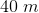. The length is ten meters more than the width. What is the area of the rectangle?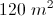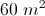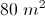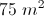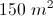Explanation:

Given a rectangle, the general equation for the perimeter is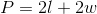and area is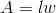whereis the length and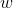is the width.

Let= width and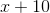= length

So the equation to solve becomes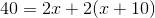sothus the width is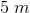and the length is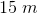.

Thus the area is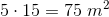### Example Question #1 : How To Find The Area Of A Rectangle

Which of the following information would not be sufficient to find the area of a rectangle?

The lengths of one side and a diagonal.

The lengths of one pair of adjacent sides.

All of the other choices list information that would be sufficient.

The perimeter and the length of one side.

The lengths of one pair of opposite sides.

The lengths of one pair of opposite sides.

Explanation:

The area of a rectangle can be calculated by multiplying the lengths of two adjacent sides. All of the choices given lists sufficient information, with one exception. We examine each of the choices.

The lengths of one pair of adjacent sides: This choice is false, as is directly stated above.

The perimeter and the length of one side: Using the perimeter formula, you can find the length of an adjacent side, making this choice false.

The lengths of one side and a diagonal: using the Pythagorean Theorem, you can find the length of an adjacent side, making this choice false.

The lengths of one pair of opposite sides: this gives you no way of knowing the lengths of the adjacent sides. This is the correct choice.

### Example Question #3 : How To Find The Area Of A Rectangle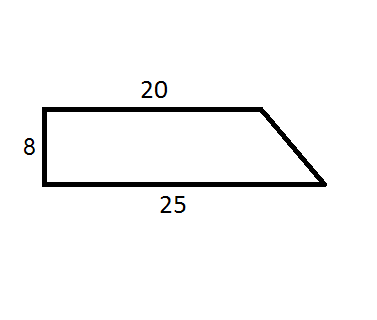Find the area of the polygon.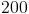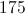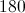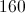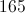Explanation:

Drawing a vertical line at the end of the side of lengthdivides the shape into a rectangle and a right triangle.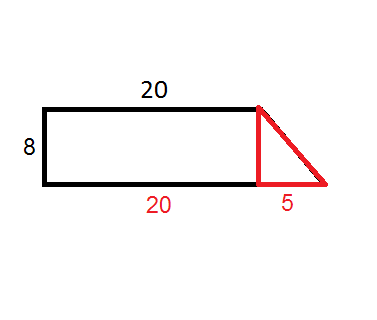The sum of the areas of the two shapes is the area of the polygon. Multiply the length of the rectangle by its width to find the area of the rectangle, and use the formula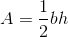, whereis the base andis the height of the triangle, to find the area of the triangle. Adding them together gives the answer.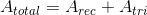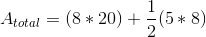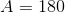### Example Question #4 : How To Find The Area Of A Rectangle

One side of a rectangle is 7 inches and another is 9 inches.  What is the area of the rectangle in inches?Explanation:

To find the area of a rectangle, multiply its width by its height. If we know two sides of the rectangle that are different lengths, then we have both the height and the width.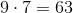### Example Question #5 : How To Find The Area Of A Rectangle

What is the area of the rectangle in the diagram?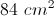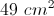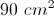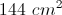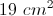Explanation:

The area of a rectangle is found by multiplying the length by the width.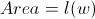The length is 12 cm and the width is 7 cm.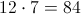Therefore the area is 84 cm2.

### Example Question #6 : How To Find The Area Of A Rectangle

What is the area of a rectangle whose length and width isinches andinches, respectively?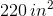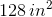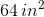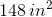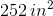Explanation:

The area of any rectangle with length,and width,is: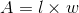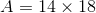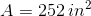### Example Question #7 : How To Find The Area Of A Rectangle

What is the area of a rectangle that has a length ofand a width of?Explanation:

Recall how to find the area of a rectangle: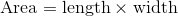Now, plug in the given length and width to find the area.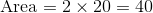### Example Question #8 : How To Find The Area Of A Rectangle

What is the area of a rectangle that has a length ofand a width of?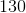Explanation:

Recall how to find the area of a rectangle:Now, plug in the given length and width to find the area.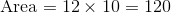### Example Question #9 : How To Find The Area Of A Rectangle

What is the area of a rectangle that has a length ofand a width of?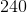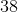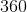Explanation:

Recall how to find the area of a rectangle:Now, plug in the given length and width to find the area.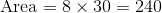### Example Question #10 : How To Find The Area Of A Rectangle

What is the area of a rectangle that has a length ofand a width of?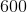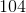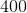Explanation:

Recall how to find the area of a rectangle:Now, plug in the given length and width to find the area.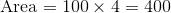← Previous 1 3 4 5 6 7 8 9 10 11